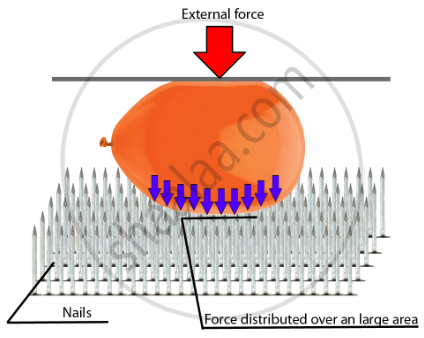# Thrust and Pressure

#### notes

Thrust and Pressure:

• The force acting on an object perpendicular to the surface is known as thrust.
• The force acting on an unit area of a surface is known as pressure.

"Pressure" = "Force" / "Area"

• The SI Unit of thrust is N/m2. It is called pascal(Pa).
• Since, pressure is indirectly proportional to the surface area of the object, thus, pressure increases with decrease in surface area and decreases with increase in surface area.
• Pressure applied on a fluid is transmitted in all directions.

#### notes

Introduction:

Fluids can be defined as any substance which is capable of flowing.

• They don’t have any shape of their own.
• For example -water does not have its own shape but it takes the shape of the container in which it is poured. But when we pour water in a tumbler it takes the shape of the tumbler
• Both liquids and gases can be categorized as fluids as they are capable of flowing.
• The volume of solids, liquids, and gas depends on the stress or pressure acting on it.

• In this chapter, we will study if we apply force on the fluid how does it affect the internal properties of fluids.

• Fluids offer very little resistance to shear stress.

• We will also study some characteristic properties of fluids.

Pressure:

The pressure is defined as force per unit area.

• "Pressure" = "Force"/"Area"

• In the CGS system, the unit of pressure is dyne cm-2 in SI, the unit of pressure is Nm-2 or pascal(pa). 1Pa = 1Nm-2

For Example:-

• Consider a very sharp needle that has a small surface area and consider a pencil whose back is very blunt and has more surface area than the needle.

• If we poke a needle in our palm it will hurt as the needle gets pierced inside our skin. Whereas if we poke the blunt side of the pencil into our hand it won’t pain so much.

• This is because the area of contact between the palm and the needle is very small therefore the pressure is large.

• Whereas the area of contact between the pencil and the palm is more therefore the pressure is less.

Conclusion: Two factors which determine the magnitude of the pressure are:-

• Force – greater the force greater is the pressure and vice-versa.

• Coverage area –greater the area less is the pressure and vice-versa.

Example:-

• Consider a balloon kept on the bed of nails and an external force is applied to it which means there are large numbers of nails on any rectangular slab. All the nails are identical and equal in height.

• We can see that the balloon does not burst. This is because there is a large number of nails and all the nails are closely spaced with each other.

• All the small pointed nails make large surface area therefore the weight of the balloon is compensated by the entire area of all the nails.

• The surface area increases therefore pressure is reduced.

• But even if one nail is greater than the others then the balloon would burst. Because then the surface area will be less as a result pressure will be more.#### description

• Thrust
• Unit of thrust
• Example of thrust
• Pressure
• Unit of Pressure
• Examples of Pressure in our daily life
If you would like to contribute notes or other learning material, please submit them using the button below.

### Shaalaa.com

Introduction [01:02:28]
S
0%